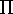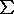SHAZAM Geometric Mean

## Calculating a Geometric Mean

Consider a sample of positive numbers x1, x2, ... xn. The geometric mean is:

Gn = (in=1 xi ) 1/n

Let An be the arithmetic mean. A result from calculus is:

GnAn

Gn = An if and only if x1 = x2 = ... = xn.

The logarithm of the geometric mean is the arithmetic mean of the log transformed data:

log(Gn) =in=1 log(xi) / n

The geometric mean is an appropriate measure of central tendency when averages of rates or index numbers are required.

For example, suppose that, in three successive years the return on an investment is 5%, 20% and `-`4%. The average rate of return can be found as the geometric mean:

((1.05)(1.20)(0.96))1/3 = 1.065

Therefore, the average rate of return (compound annual growth rate) is 6.5%.

#### Example

This example is from J. Freund, F. Williams, B. Perles and C. Sullivan [Modern Business Statistics, Prentice-Hall, 1969, Chapter 16]. Price indexes are available for seven food commodities. The indexes express the 1968 price as a percentage of the 1956 price.

The SHAZAM commands (filename: `GMEAN.SHA`) that compute and compare the arithmetic mean with the geometric mean are given below. The geometric mean is calculated from the arithmetic mean of the log transformed data.

 ```SAMPLE 1 7 READ PRICE / BYVAR LIST 137 146 163 98 144 292 119 * Calculate the arithmetic mean and median STAT PRICE / MEAN=AN PMEDIAN * Calculate the geometric mean GENR LPRICE=LOG(PRICE) STAT LPRICE / MEAN=LGN GEN1 GN=EXP(LGN) PRINT AN GN STOP ```

The SHAZAM output can be viewed. The output shows the following results:

 Arithmetic Mean 157 Median 144 Geometric Mean 149[SHAZAM Guide home]

#### SHAZAM output

```|_SAMPLE 1 7
1 VARIABLES AND        7 OBSERVATIONS STARTING AT OBS       1

PRICE
137.0000       146.0000       163.0000       98.00000       144.0000
292.0000       119.0000

|_* Calculate the arithmetic mean and median
|_STAT PRICE / MEAN=AN PMEDIAN
NAME        N   MEAN        ST. DEV      VARIANCE     MINIMUM      MAXIMUM
PRICE        7   157.00       63.082       3979.3       98.000       292.00

VARIABLE = PRICE
MEDIAN =    144.00
LOWER 25%=   119.00     UPPER 25%=   163.00     INTERQUARTILE RANGE=  44.00
MODE NOT APPLICABLE

|_* Calculate the geometric mean
|_GENR LPRICE=LOG(PRICE)
|_STAT LPRICE / MEAN=LGN
NAME        N   MEAN        ST. DEV      VARIANCE     MINIMUM      MAXIMUM
LPRICE       7   5.0011      0.34044      0.11590       4.5850       5.6768
|_GEN1 GN=EXP(LGN)

|_PRINT AN GN
AN
157.0000
GN
148.5828
|_STOP
```[SHAZAM Guide home]